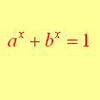#### You may also like### Growing

Which is larger: (a) 1.000001^{1000000} or 2? (b) 100^{300} or 300! (i.e.factorial 300)### What Do Functions Do for Tiny X?

Looking at small values of functions. Motivating the existence of the Taylor expansion.### Equation Attack

The equation a^x + b^x = 1 can be solved algebraically in special cases but in general it can only be solved by numerical methods.

# Building Approximations for Sin(x)

##### Age 16 to 18Challenge Level

In general, any function whose derivatives of all orders are finite can be written as a power series

$$f(x) = f(0) + x f'(0) + \frac{x^2}{2!}f''(0) + \dots + \frac{x^n}{n!}f^{(n)}(0)+\dots$$
This expansion is an infinite series (not a polynomial). Truncating this series at a given point provides us with a polynomial approximation to f(x).

The question of how big the errors are in this approximation is a difficult one to answer, and more details will be discovered at university in Numerical Analysis courses.